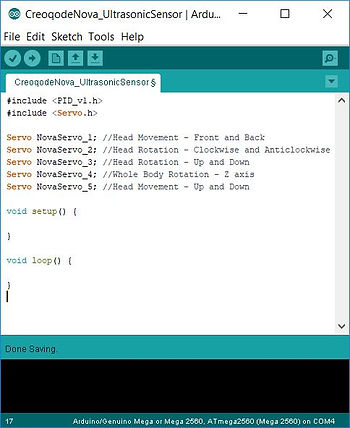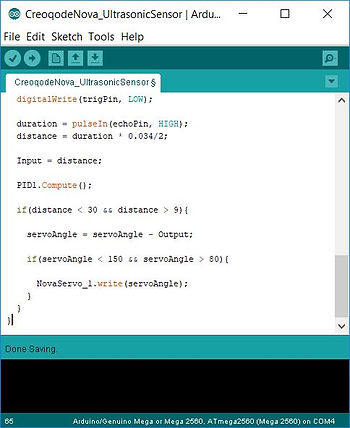top of page## ULTRASONIC SOUND SENSOR | HC-SR04HC-SR04 is an ultrasonic sound sensor. This sensor provides 2 cm to 400 cm of non-contact measurement functionality with a ranging accuracy that can reach up to 3 mm. It includes an ultrasonic transmitter, an ultrasonic receiver and a control circuit.

We will be using this sensor as a fun way to interact with Nova. In this chapter, we are going to program Nova to keep 15 cm distance from an object in front of its face. When you move the object closer or far away, Nova will move backward and forward in order to keep the same distance of 15 cm between itself and the object.HC-SR04 ultrasonic sound sensor has only 4 pins to connect: VCC, Trig, Echo and GND. By using the jumper wires provided in the kit, connect VCC to VCC on Servo Shield, GND to GND, Trig to 46, Echo to 44. You can attach Female - Female jumper wires with Male - Female jumper wires in order to achieve the necessary length for connections.The working principle of this sensor is very simple. The circular module on the left in the photo above (the one on top when looking on Nova's face) is the trigger module which sends out ultrasonic sound waves, that human ear cannot hear. The circular module on the right (the one on bottom when looking on Nova's face) is the receiver module. An ultrasonic sound wave is sent out from the trigger module, the same sound wave hits a surface in front of the sensor, it comes back after reflecting from that surface, and it is received by the receiver module. The time difference between the sound wave is sent out and received back is used to measure the distance between the sensor and the object/surface, since the velocity of an ultrasonic sounds wave is already known.

Since you have completed the connections, let's open Arduino Software (IDE) and start building our sketch. Let's start by including servo and PID libraries as below:

#include <PID_v1.h>
#include <Servo.h>

Then, define all the servos of Nova. For this tutorial, we will only be using one servo, but let's stick to the template and define all 5 of them.

Servo NovaServo_1; //Head Movement - Front and Back
Servo NovaServo_2; //Head Rotation - Clockwise and Anticlockwise
Servo NovaServo_3; //Head Rotation - Up and Down
Servo NovaServo_4; //Whole Body Rotation - Z axis
Servo NovaServo_5; //Head Movement - Up and DownNow, we need define which pins Trig and Echo are connected to on Servo Shield, thus on Creoqode Mini Mega. We also have to create 3 variables: duration for the time difference between trigger and receiver, distance for the calculated distance based on duration, and servoAngle for the shaft angle of the servo that will be rotated based on the distance calculated. We are initialising servoAngle as 110 degrees, as this will be the starting position of this application.

const int trigPin = 46;
const int echoPin = 44;

long duration;
int distance;
int servoAngle = 110;

Following the above lines, we are setting up our PID controller. We are defining Input, Setpoint and Output variables. Then, Kp, Ki and Kd constants are defined and assigned with specific variables. The constant values of 0.51, 1.1 and 0, for Kp, Ki and Kd respectively, are found after some time of trials done with the Nova in our lab. These numbers might require some slight tuning for your Nova, as there will never be one perfect tuning for all cases. Give it a try and see if Nova needs adjusting K constants. And finally, we are creating our PID function called PID1.

double Setpoint, Input, Output;

double Kp = 0.51;
double Ki = 1.1;
double Kd = 0;

PID PID1(&Input, &Output, &Setpoint, Kp, Ki, Kd, DIRECT);Since we have defined our variables and created the PID function, now it is time to create the setup() function. For this one, we have to define Trig pin as an output (this pin will be sending out the signal for the sound wave) and Echo pin as an input (this pin will be receiving the signal from the sound wave). Then, we have to set up the serial function at baud rate of 9600, we might want to see the distance between the object and Nova through the Serial Monitor of Arduino Software (IDE). Following this, we need to define which pin that the servo we are going to use is attached to, and initialise the position of the servo by adjusting its shaft angle to servoAngle variable.

pinMode(trigPin, OUTPUT);
pinMode(echoPin, INPUT);

Serial.begin(9600);

NovaServo_1.attach(32);
NovaServo_1.write(servoAngle);

We now need to set up the PID function. First, we initialise the function by SetMode(). Then, SetSampleTime() function determines the interval between each PID computation in milliseconds. SetOutputLimits() function determines the maximum and minimum values that PID function can generate as Output. It is a very important function, especially for robotics projects, because due to the noise or some inaccuracies in the system, PID can generate instantaneous large values that can bring the system to an unstable state. We have set the minimum and maximum values to -3 and 3 degrees, so even if there is huge error gap between the Input and Setpoint, the value of Output will not be exceeding these.

Finally, we are defining the Setpoint to be 15, as we want the distance between Nova and the object/surface to be 15 cm for this project. You can change it as you wish. And we are assigning the calculated distance to the Input variable. PID will be working based on the difference between Setpoint which is 15 cm and Input which is the current distance measured by the sensor.

PID1.SetMode(AUTOMATIC);
PID1.SetSampleTime(10);
PID1.SetOutputLimits(-3, 3);

Setpoint = 15;
Input = distance;Now, let's create our loop() function. First of all, we need to send out an ultrasonic sound wave and receive it at each iteration of the loop() function. We make sure the Trig pin is connected to GND by setting it to LOW, then we set it to HIGH to send the signal, and then again we set it to LOW. For the Echo pin, by using the pulseIn() function, we are receiving the signal of the ultrasonic sound wave that is reflected from the object/surface accross. Then, by using the duration in the formula, we calculate the distance.

digitalWrite(trigPin, LOW);
delayMicroseconds(2);
digitalWrite(trigPin, HIGH);
delayMicroseconds(10);
digitalWrite(trigPin, LOW);

duration = pulseIn(echoPin, HIGH);
distance = duration * 0.034/2;At this stage, since we have assigned a value for Setpoint (the target value) and calculated the current distance between Nova and the object, we can initiate the PID controller by using Compute() function. Now, we are updating servoAngle's value by subtracting the generated Output from servoAngle's previous value. However, we are doing this step with an "if" condition. servoAngle is only updated if the calculated distance is betwen 30 cm and 9 cm. Any objects detected out of this range will not be considered, and Nova will not be moving.

Then, we are using servo.write() function to move Nova with the current value of updated servoAngle. This step is also iterated with an "if" condition. Servo is only moved if the servoAngle's value is between 80 and 150, as this is the safe movement range of Nova Servo 1, which is connected to digital pin 32.

Input = distance;

PID1.Compute();

if(distance < 30 && distance > 9){
servoAngle = servoAngle - Output;
if(servoAngle < 150 && servoAngle > 80){
NovaServo_1.write(servoAngle);
}
}That's it! Now, upload your sketch to Nova and test it. Instead of a random shaped object, flat surfaces work much better with this sensor as the sound waves are reflected without changing much direction.

bottom of page